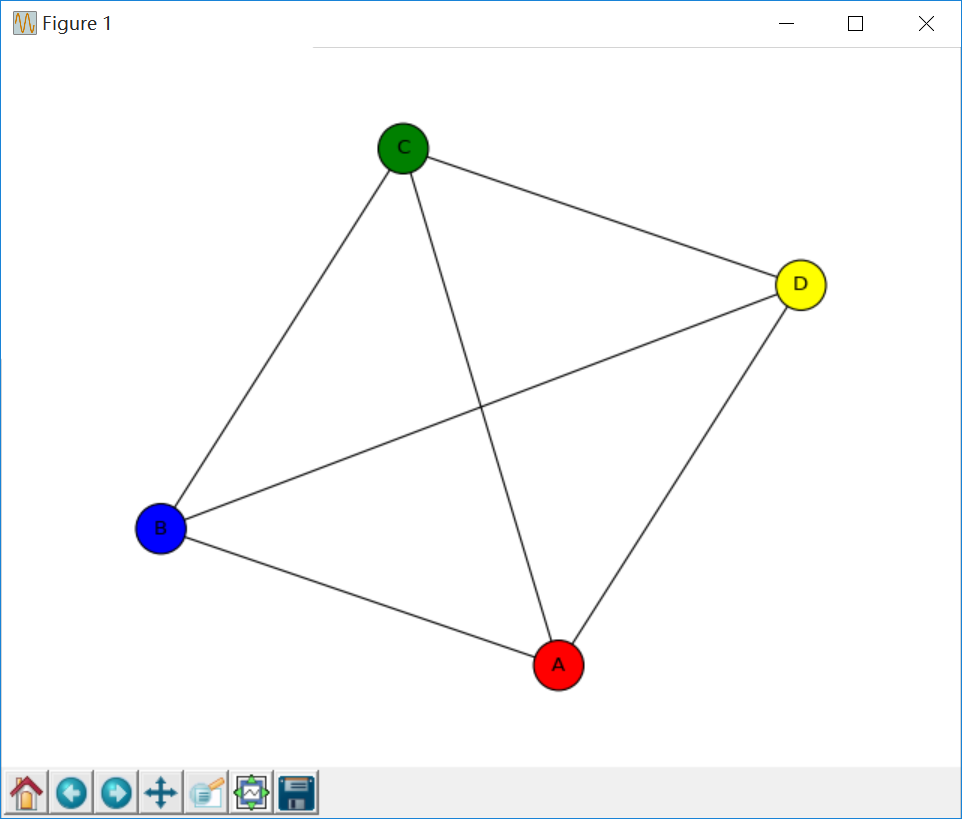# [Pyhon大数据分析] 五.人民网新闻话题抓取及Gephi构建主题知识图谱### 文章目录# 一.人民网数据抓取

http://society.people.com.cn/GB/369130/431577/431608/index.html• 标题：titles = driver.find_elements_by_xpath(’//div[@class=" p2j_list_lt fl"]/ul/li’)
• 链接：links = driver.find_elements_by_xpath(’//div[@class=" p2j_list_lt fl"]/ul/li/a’)• zw = soup.find(attrs={“class”:“box_con”})# -*- coding: utf-8 -*-
import os
import re
import csv
import time
import json
import random
import urllib.request
from lxml import etree
from bs4 import BeautifulSoup
from selenium import webdriver
from selenium.webdriver.chrome.options import Options

#-------------------------------------------------写入文件-------------------------------------------------
path = os.getcwd() + "/yqkx_data.csv"
csvfile = open(path, 'a', newline='', encoding = 'utf-8-sig')
writer = csv.writer(csvfile)
writer.writerow(('序号','文章标题','发布时间','文章链接','文章内容'))

#--------------------------------------------YQ快讯-数据抓取---------------------------------------------
url = "http://society.people.com.cn/GB/369130/431577/431608/index.html"
driver = webdriver.Chrome() #chromedriver.exe置于python37根目录
driver.implicitly_wait(5)
chrome_option = webdriver.ChromeOptions()
driver.get(url) #打开网页网页
driver.implicitly_wait(6) #等待加载六秒

#-------------------------------------------------获取标题-------------------------------------------------
titles = driver.find_elements_by_xpath('//div[@class=" p2j_list_lt fl"]/ul/li')
for t in titles:
print(t.text)

print("\n\n==========================================================")

#-------------------------------------------------获取正文-------------------------------------------------
def get_content(url):
print(url)
try:
soup = BeautifulSoup(content,"html.parser")
#来源
ly = soup.find(attrs={"class":"fl"}).get_text()
#print(ly)
#正文
zw = soup.find(attrs={"class":"box_con"})
#防止某些文章仅图片
if zw is not None:
zw = zw.get_text()
zw = zw.replace("\n", "")
else:
zw = ""
print(zw)
print("succeed")
return ly,zw
except Exception as e:
zw = e.partial
ly = ""
print("except")
print(zw)
return ly, page

#-------------------------------------------------写入文件-------------------------------------------------
k = 0
while k<len(titles):
#序号
num = str(k+1)
#文章标题和发布时间
value = titles[k].text.split('\n')
con_title = value
con_time = value
#文件链接
#获取来源和正文
ly,zw = get_content(url)
content = (num, con_title, con_time, url, zw)
#文件写入操作
writer.writerow((content))
k = k + 1

#文件关闭
csvfile.close()# 二.文本关键词提取# coding=utf-8
import jieba
import re
import time
from collections import Counter
from snownlp import SnowNLP

#------------------------------------中文分词------------------------------------
cut_words = ""
all_words = ""
f = open('all-data-key.txt', 'w', encoding='utf-8')
for line in open('all-data.txt', encoding='utf-8'):
line = line.strip('\n')
#停用词过滤
line = re.sub('[0-9’!"#\$%&\'()*+,-./:;<=>?@，。?★、…【】《》？“”‘’！[\\]^_{|}~\s]+', "", line)
seg_list = jieba.cut(line, cut_all=False)
cut_words = (" ".join(seg_list))

#计算关键词
all_words = cut_words.split()
c = Counter()
for x in all_words:
if len(x)>1 and x != '\r\n':
c[x] += 1
#Top50
output = ""
#print('\n词频统计结果：')
for (k,v) in c.most_common(50):
#print("%s:%d"%(k,v))
output += k + " "

f.write(output+"\n")
else:
f.close()# 三.共现矩阵

文章标题                                        作者

Python网络爬虫                                 A，D，C



(1) 首先写代码抓取该领域文章的所有作者或关键词，即：A、B、C、D。
(2) 接着获取对应的共现矩阵，比如文章“大数据发展现状分析”，则认为A、B、C共现，在他们之间建立一条边。共现矩阵如下所示：

$\left[ \begin{matrix} -& A & B & C & D \\ A & 0 & 1 & 2 & 1 \\ B & 1 & 0 & 1 & 1 \\ C & 2 & 1 & 0 & 1 \\ D & 1 & 1 & 1 & 0 \end{matrix} \right] \tag{1}$

(3) 通过共现矩阵分别获取两两关系及权重，再写入CSV或Excel文件中，如下所示。

$\left[ \begin{matrix} Source & Target & Weight \\ A & B & 1 \\ A & C & 2 \\ A & D & 1 \\ B & C & 1 \\ B & D & 1\\ C & D & 1 \end{matrix} \right] \tag{2}$

(4) 将该CSV文件导入Gephi中，并绘制相关的图形。因为该实例节点比较少，下面是Pyhton调用Networkx绘制的代码及图形。

# -*- coding: utf-8 -*-
import networkx as nx
import matplotlib.pyplot as plt

#定义有向图
DG = nx.Graph()
#添加五个节点(列表)
print(DG.nodes())
#添加边(列表)
#DG.add_edges_from([('A', 'B'), ('A', 'C'), ('A', 'D'), ('B','C'),('B','D'),('C','D')])
print(DG.edges())
#绘制图形 设置节点名显示\节点大小\节点颜色
colors = ['red', 'green', 'blue', 'yellow']
nx.draw(DG,with_labels=True, node_size=900, node_color = colors)
plt.show()# 四.主题关键词共现分析

# -*- coding: utf-8 -*-
"""
@author: eastmount CSDN 2020-04-12
"""
import pandas as pd
import numpy as np
import codecs
import networkx as nx
import matplotlib.pyplot as plt
import csv
from scipy.sparse import coo_matrix

#---------------------------第一步:读取数据-------------------------------
word = [] #记录关键词
f = open("all-data-key.txt", encoding='utf-8')
while line:
#print line
line = line.replace("\n", "") #过滤换行
line = line.strip('\n')
for n in line.split(' '):
#print n
if n not in word:
word.append(n)
f.close()
print(len(word)) #关键词总数

#--------------------------第二步 计算共现矩阵----------------------------
a = np.zeros([2,3])
print(a)

#共现矩阵
#word_vector = np.zeros([len(word),len(word)], dtype='float16')

#MemoryError：矩阵过大汇报内存错误
#采用coo_matrix函数解决该问题
print(len(word))
#类型<type 'numpy.ndarray'>
word_vector = coo_matrix((len(word),len(word)), dtype=np.int8).toarray()
print(word_vector.shape)

f = open("all-data-key.txt", encoding='utf-8')
while line:
line = line.replace("\n", "") #过滤换行
line = line.strip('\n') #过滤换行
nums = line.split(' ')

#循环遍历关键词所在位置 设置word_vector计数
i = 0
j = 0
while i<len(nums):         #ABCD共现 AB AC AD BC BD CD加1
j = i + 1
w1 = nums[i]           #第一个单词
while j<len(nums):
w2 = nums[j]       #第二个单词
#从word数组中找到单词对应的下标
k = 0
n1 = 0
while k<len(word):
if w1==word[k]:
n1 = k
break
k = k +1
#寻找第二个关键字位置
k = 0
n2 = 0
while k<len(word):
if w2==word[k]:
n2 = k
break
k = k +1
#重点: 词频矩阵赋值 只计算上三角
if n1<=n2:
word_vector[n1][n2] = word_vector[n1][n2] + 1
else:
word_vector[n2][n1] = word_vector[n2][n1] + 1
#print n1, n2, w1, w2
j = j + 1
i = i + 1
#读取新内容
f.close()

#--------------------------第三步  TXT文件写入--------------------------
res = open("word_word_weight.txt", "a+", encoding='utf-8')
i = 0
while i<len(word):
w1 = word[i]
j = 0
while j<len(word):
w2 = word[j]
#判断两个词是否共现 共现&词频不为0的写入文件
if word_vector[i][j]>0:
#print w1 +" " + w2 + " "+ str(int(word_vector[i][j]))
res.write(w1 +" " + w2 + " "+ str(int(word_vector[i][j]))  +  "\n")
j = j + 1
i = i + 1
res.close()

#--------------------------第四步  CSV文件写入--------------------------
c = open("word-word-weight.csv","w", encoding='utf-8', newline='')    #解决空行
#c.write(codecs.BOM_UTF8)                                 #防止乱码
writer = csv.writer(c)                                    #写入对象
writer.writerow(['Word1', 'Word2', 'Weight'])

i = 0
while i<len(word):
w1 = word[i]
j = 0
while j<len(word):
w2 = word[j]
#判断两个词是否共现 共现词频不为0的写入文件
if word_vector[i][j]>0:
#写入文件
templist = []
templist.append(w1)
templist.append(w2)
templist.append(str(int(word_vector[i][j])))
#print templist
writer.writerow(templist)
j = j + 1
i = i + 1
c.close()


5267
[[0. 0. 0.]
[0. 0. 0.]]
5267
(5267, 5267)
`# 五.Gephi绘制主题知识图谱

## 1.数据准备## 2.导入数据## 3.调整参数绘制图谱## 4.图谱优化# 六.总结(By:Eastmount 2020-04-12 周末下午5点于贵阳 http://blog.csdn.net/eastmount/)

#### Python小白也会用的pandas用法总结

07-05

03-212万+

#### [Pyhon疫情大数据分析] 四.微博话题抓取及新冠肺炎疫情文本挖掘和情感分析06-19153

#### AI+GIS实现网络新闻舆情地理分布可视化

12-111万+

#### 利用Python+Gephi构建金庸人物知识图谱

03-071万+

#### [Pyhon疫情大数据分析] 三.新闻信息抓取及词云可视化、文本聚类和LDA主题模型文本挖掘

03-061万+

#### 中国疫情发展趋势分析预测（python实现）

06-20220

#### Gephi教程——外观和布局

04-054613

#### Python爬虫学习分享(疫情数据爬取＋可视化)

09-026313

#### [Python知识图谱] 四.Python和Gephi实现中国知网合作关系知识图谱

02-013889

#### Gephi|交通出行可视化

02-21294

#### 全网独家---【科学知识图谱】软件，做知识图谱的利器

05-111万+

#### Python 网络爬虫实战：爬取人民日报新闻文章

02-281万+

#### 使用networkx导出关系网络并用gephi进行简单分析

12-175841

#### [关系图谱] 二.Gephi导入共线矩阵构建作者关系图谱

02-172万+

#### [Pyhon疫情大数据分析] 一.腾讯实时数据爬取、Matplotlib和Seaborn可视化分析全国各地区、某省各城市、新增趋势

05-0483

#### 用主题模型可视化分析911新闻（Python版）©️2020 CSDN 皮肤主题: 书香水墨 设计师: CSDN官方博客点击重新获取扫码支付1.余额是钱包充值的虚拟货币，按照1:1的比例进行支付金额的抵扣。
2.余额无法直接购买下载，可以购买VIP、C币套餐、付费专栏及课程。余额充值